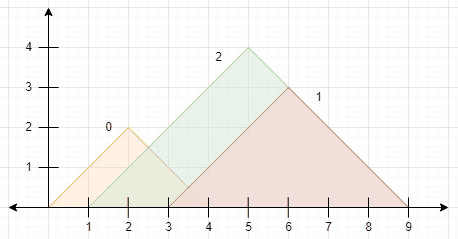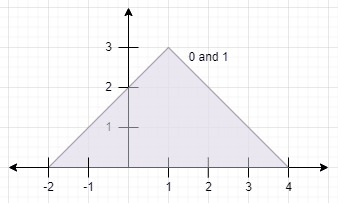##### Welcome to Subscribe On Youtube

Formatted question description: https://leetcode.ca/all/2345.html

# 2345. Finding the Number of Visible Mountains

## Description

You are given a 0-indexed 2D integer array peaks where peaks[i] = [xi, yi] states that mountain i has a peak at coordinates (xi, yi). A mountain can be described as a right-angled isosceles triangle, with its base along the x-axis and a right angle at its peak. More formally, the gradients of ascending and descending the mountain are 1 and -1 respectively.

A mountain is considered visible if its peak does not lie within another mountain (including the border of other mountains).

Return the number of visible mountains.

Example 1:Input: peaks = [[2,2],[6,3],[5,4]]
Output: 2
Explanation: The diagram above shows the mountains.
- Mountain 0 is visible since its peak does not lie within another mountain or its sides.
- Mountain 1 is not visible since its peak lies within the side of mountain 2.
- Mountain 2 is visible since its peak does not lie within another mountain or its sides.
There are 2 mountains that are visible.

Example 2:Input: peaks = [[1,3],[1,3]]
Output: 0
Explanation: The diagram above shows the mountains (they completely overlap).
Both mountains are not visible since their peaks lie within each other.


Constraints:

• 1 <= peaks.length <= 105
• peaks[i].length == 2
• 1 <= xi, yi <= 105

## Solutions

• class Solution {
public int visibleMountains(int[][] peaks) {
int n = peaks.length;
int[][] arr = new int[n];
Map<String, Integer> cnt = new HashMap<>();
for (int i = 0; i < n; ++i) {
int x = peaks[i], y = peaks[i];
arr[i] = new int[] {x - y, x + y};
cnt.merge((x - y) + "" + (x + y), 1, Integer::sum);
}
Arrays.sort(arr, (a, b) -> a == b ? b - a : a - b);
int ans = 0;
int cur = Integer.MIN_VALUE;
for (int[] e : arr) {
int l = e, r = e;
if (r <= cur) {
continue;
}
cur = r;
if (cnt.get(l + "" + r) == 1) {
++ans;
}
}
return ans;
}
}

• class Solution {
public:
int visibleMountains(vector<vector<int>>& peaks) {
vector<pair<int, int>> arr;
for (auto& e : peaks) {
int x = e, y = e;
arr.emplace_back(x - y, -(x + y));
}
sort(arr.begin(), arr.end());
int n = arr.size();
int ans = 0, cur = INT_MIN;
for (int i = 0; i < n; ++i) {
int l = arr[i].first, r = -arr[i].second;
if (r <= cur) {
continue;
}
cur = r;
ans += i == n - 1 || (i < n - 1 && arr[i] != arr[i + 1]);
}
return ans;
}
};

• class Solution:
def visibleMountains(self, peaks: List[List[int]]) -> int:
arr = [(x - y, x + y) for x, y in peaks]
cnt = Counter(arr)
arr.sort(key=lambda x: (x, -x))
ans, cur = 0, -inf
for l, r in arr:
if r <= cur:
continue
cur = r
if cnt[(l, r)] == 1:
ans += 1
return ans


• func visibleMountains(peaks [][]int) (ans int) {
n := len(peaks)
type pair struct{ l, r int }
arr := make([]pair, n)
for _, p := range peaks {
x, y := p, p
arr = append(arr, pair{x - y, x + y})
}
sort.Slice(arr, func(i, j int) bool { return arr[i].l < arr[j].l || (arr[i].l == arr[j].l && arr[i].r > arr[j].r) })
cur := math.MinInt32
for i, e := range arr {
l, r := e.l, e.r
if r <= cur {
continue
}
cur = r
if !(i < n-1 && l == arr[i+1].l && r == arr[i+1].r) {
ans++
}
}
return
}